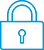cancel
Showing results for
Did you mean:It has been a while since anyone has replied. Simply ask a new question if you would like to start the discussion again.New member
1 0 0 0
Message 1 of 5
759
Flag Post

# HP Prime distanceHP Recommended
HP Prime
How to find distance between intersections of two graphs quickly? Is there a way to copy a point (x,y) into a variable to work with them?
Tags (4)
4 REPLIES 4Level 8
650 635 124 222
Message 2 of 5
Flag PostHP Recommended

Yes, you can easily store them in the complex variables Z1, Z2, etc.  Here's one way to do that which might not be the easiest way but it does work.

Assuming that you're using the Function app to graph your functions (let's say F1 and F2), and using Menu/Fcn/Intersection to find intersections, then Prime stores the X value of the most recently found intersection into the app's result variable called 'Isect'.

Therefore, after the intersection is found in Plot view, exit the Plot view by pressing Home, and type this:

Z1:=(Isect, F1(Isect))

Then press Plot and find the next intersection, then press Home and type this:

Z2:=(Isect, F1(Isect))

Your two points of intersection are now stored in Z1 and Z2. To have them displayed in the usual point notation, press Shift Home (Settings) and set the Complex display mode to (a,b).

The absolute distance between Z1 and Z2 of course is:

ABS(Z1-Z2)

A shortcut for ABS( ) is the absolute-value symbols on the Shift change-sign key: |Z1-Z2|.

Note: This is WAY more complicated than it is on the HP 50g, which puts the intersection POINTS on the stack automatically (not just their X values), so that the user doesn't need to store them anywhere, and the difference between them can then be found in TWO keystrokes.  So I suspect that there's an easier way to do it on the Prime than the way I described above.  Anybody here know what it is?  If so, please share it!

Disclaimer: I don't work for HP. I just love using their calculators.

-Joe-Level 6
305 297 32 76
Message 3 of 5
Flag PostHP Recommended

Hello Joe,

It can be done a bit easier.

Instead of using Isect you can simply use X.

That is because the x-coordinate of the cursor in Plot view is stored into X after leaving Plot view.

Even when you do nothing in Plot view, when leaving it, X will generally be changed.

Using the Intersection function automatically puts the cursor on the intersection and so changes X.

You could also tap the screen at the position of the intersection to change the x-coordinate and so X.

Because zooming in and out is very easy in the Prime, by simply tapping a certain position and pressing the + or - key, this can be very efficient.

In the Advanced Graphing app both X and Y will will be changed into both coordinates of the cursor.

Instead of storing the coordinates into a complex number it is also possible to store them into a vector, eg M1, which is a reserved variable which can contain both matrices and vectors.

So one could also write: M1:=[X,F1(X)]

or create a new variable: v1:=[X,F1(X)]Level 8
650 635 124 222
Message 4 of 5
Flag PostHP Recommended

Excellent suggestions! Thanks!

Your idea of using X instead of Isect suggests another idea: Use Y instead of F1(X).  This is because both X and Y are set to the point of intersection.

Therefore, after finding the intersection using the graphing functions, you can do any of the following to save the point:

Z1:=(X,Y)

M1:=[X,Y]

v1:=[X,Y]

-Joe-Level 6
305 297 32 76
Message 5 of 5
Flag PostHP Recommended

Well seen Joe.

Again a bit easier!

† The opinions expressed above are the personal opinions of the authors, not of HP. By using this site, you accept the Terms of Use and Rules of Participation